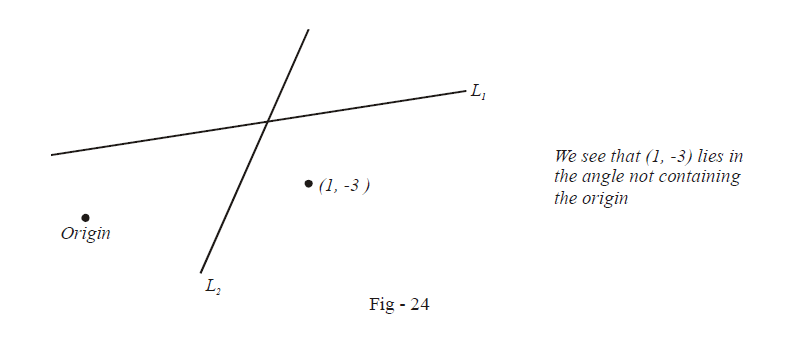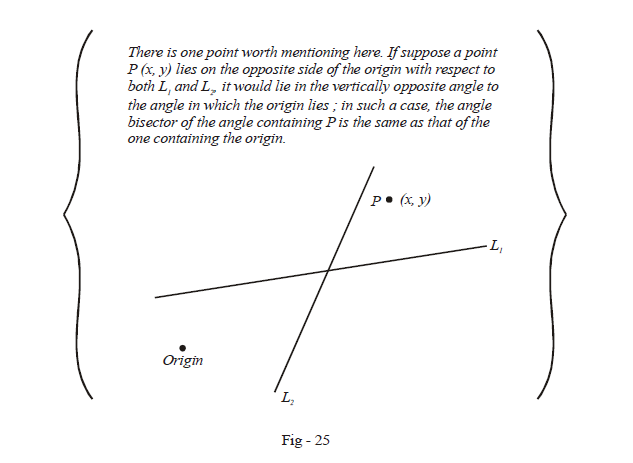# Examples on Angle Bisectors of Angles Formed by Intersecting Lines

Go back to  'Straight Lines'

Example – 13

Find the angle bisector of the angle between the straight lines $${L_1}:3x - 4y + 7 = 0\,\,\,\,{\text{and }}{L_2}:12x - 5y - 8 = 0$$ which contains the origin.

Solution: Following the discussion of the preceeding article, we first modify the equations L1 and L2 so that the constant terms in both the equations are of the same sign (say both positive):

\begin{align}&{L_1}\,\,\,\,:\,\,\,3x - 4y + 7 = 0\\&{L_2}\,\,\,\,:\,\,\, - 12x + 5y + 8 = 0\end{align}

The angle bisector of the angle containing the origin is

\begin{align}&\frac{{(3x - 4y + 7)}}{{\sqrt {{3^2} + {4^2}} }} = + \frac{{( - 12x + 5y + 8)}}{{\sqrt {{{12}^2} + {5^2}} }}\\ \Rightarrow \qquad &99x - 77y + 51=0 \end{align}

Evaluating the other angle bisector is left to the reader as an exercise.

Example – 14

Find the bisector of the angle between the lines $$x + 2y - 11 = 0$$ and $$3x - 6y - 5 = 0$$ which contains the point (1, –3).

Solution: Again we first arrange the equations of the two lines such that constant terms are positive

\begin{align}{L_1}\,\,\,\,:\,\,\, - x - 2y + 11 = 0\\{L_2}\,\,\,\,:\,\,\, - 3x + 6y + 5 = 0\end{align}

Note that

\begin{align}&{L_1}({\text{at origin}}) > 0\,\,{\rm{and }}\,{L_1}({\rm{at}}(1,\, - 3)) > 0\\\\&\qquad\quad \Rightarrow \quad\text{Origin and (1, –3) are on the same side of }{L_1} \\\\&{L_2}({\text{at origin}}) > 0\,\,{\rm{and }}\,{L_2}({\rm{at}}(1,\, - 3)) < 0\\\\&\qquad\quad\Rightarrow \quad \text{Origin and (1, –3) are on the opposite sides of }{L_2}\end{align}

This means that the point (1, –3) does not lie in the same region as the origin, since (1, –3) must be on the opposite side of the origin with respect to L2.The example figure below will make this clear:Thus, it is clear that (1, –3) lies in the angle not containing the origin.To determine the angle bisector of the angle containing (1, –3), we simply determine the angle bisector of the angle not containing the origin, i.e.

\begin{align}&\frac{{ - x - 2y + 11}}{{\sqrt 5 }} = - \frac{{ - 3x + 6y + 5}}{{3\sqrt 5 }}\\\Rightarrow \qquad &6\sqrt 5 x = 38\sqrt 5 \\\Rightarrow \qquad &x = \frac{{19}}{3}\end{align}

Note that to determine the angle bisector of the angle containing the point P as in Fig.-25, we would have chosen the angle bisector of the angle containing the origin.